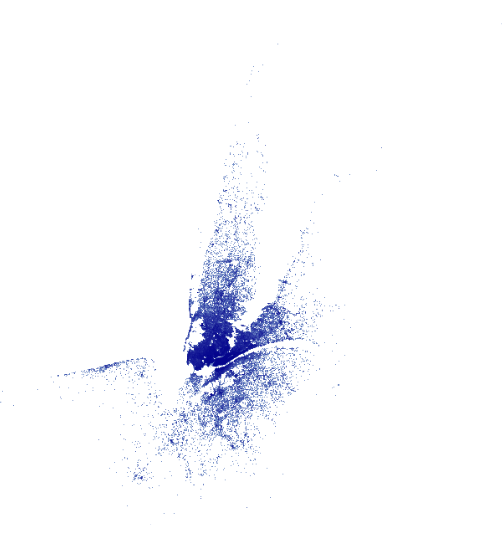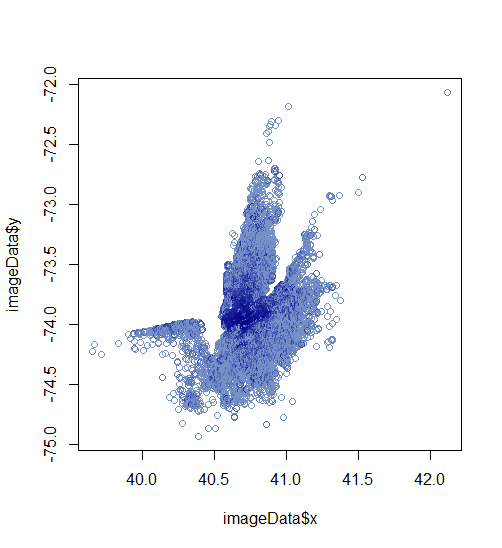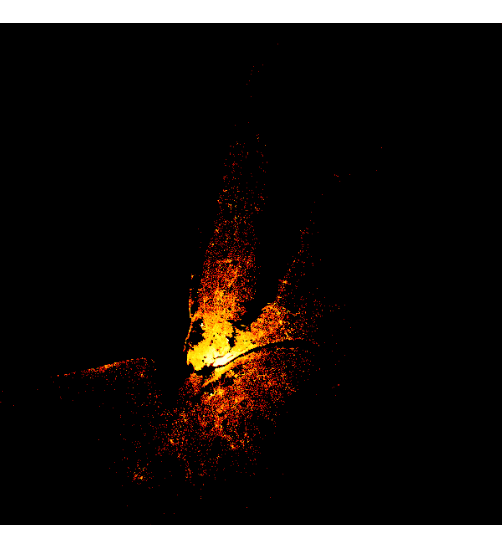# Introduction to rasterly

#### 2020-06-07

library(rasterly)
library(data.table)
library(lubridate)
library(grid)
library(plotly)

rasterly makes it easy to rapidly generate raster images for large datasets. Although the package is inspired by the Datashader library available for Python, rasterly does not attempt to reproduce all the features of Datashader. Rather, rasterly offers comparable performance to Datashader when generating rasters from source data. rasterly attempts to provide a flexible, convenient interface which should feel familiar to users of ggplot2 and its aesthetics-based approach to customizing plots and figures.

A raster may be described as a matrix of cells or pixels arranged in grid-like fashion, in which each pixel represents a value in the source data. When combined with the package and Plotly.js, rasterly enables analysts to generate interactive figures with very large datasets which are responsive enough to embed into Dash for R applications.

The rasterly function creates a rasterly object, to which aggregation layers may be added. This function is the first step in the process of generating raster image data using the package. The rasterly function is not intended to be used in isolation, since aggregation layers are required for full functionality.

## Data set

The dataset used in this vignette describes Uber trips taken in New York City from April 1st to September 30th of 2014.

# Load data
ridesRaw_1 <- "https://raw.githubusercontent.com/plotly/datasets/master/uber-rides-data1.csv" %>%
ridesRaw_2 <- "https://raw.githubusercontent.com/plotly/datasets/master/uber-rides-data2.csv" %>%
ridesRaw_3 <- "https://raw.githubusercontent.com/plotly/datasets/master/uber-rides-data3.csv"  %>%
ridesDf <- list(ridesRaw_1, ridesRaw_2, ridesRaw_3) %>%
data.table::rbindlist()

# Extract hour of trip taken
time <- lubridate::ymd_hms(ridesDf$Date/Time) ridesDf <- ridesDf[, 'Date/Time':=NULL][, list(Lat, Lon, hour = lubridate::hour(time), month = lubridate::month(time), day = lubridate::day(time))] This dataset has 4,533,327 observations, and includes the variables “latitude”, “longitude”, “hour”, “month” and “day”. ## Basic If we were to use graphics::plot(), it would take several minutes to render the image. What if we “rasterized” the image instead? start_time <- Sys.time() p <- ridesDf %>% rasterly(mapping = aes(x = Lat, y = Lon)) %>% rasterly_points() p end_time <- Sys.time() end_time - start_timeA raster image, in essence, is a large sparse matrix and each element is a hexadecimal color (A character vector with elements of 7 or 9 characters, “#” followed by the red, blue, green and optionally alpha values). Since the range (x, y) of this display can be acquired, we can also map the image into a data.frame with mapping coordinates x, y and color. For example: image <- as.raster(matrix((1:4)/4, nrow = 2)) image ## [,1] [,2] ## [1,] "#404040" "#BFBFBF" ## [2,] "#808080" "#FFFFFF" # mapping this image onto a 1 <= x <= 2 and 2 <= y <= 5 plane rasterly::image2data(image, x_range = c(1, 2), y_range = c(2, 5)) ## x y color ## 1: 1 5 #404040 ## 2: 1 2 #808080 ## 3: 2 5 #BFBFBF ## 4: 2 2 #FFFFFF In this way, we can transform a large dataset (million or billion) into a raster image (say 400 $$\times$$ 400), then, mapping this image to a data.frame but dropping blank ones. So the size can decrease from 160000 to rough 30k or 40k (It really depends on data). In other word, we can always reduce a huge size to a reasonable size and the darkness of color is judged by the “Reduction Function” (see section ‘API’ for more info) imageData <- rasterly::image2data(p) # basic graphics # It is slow but still much faster than drawing the huge data directly) plot(x = imageData$x, y = imageData$y, col = imageData$color)## rasterly Structure

#### Subsetting

rasterly() generates a parent layer containing initial settings to generate the raster, which include plot_height, plot_width among others; child layers such as rasterly_points() can be piped in as well. Note that “p” above is a list of environments. The elements in “p” can be easily extracted or replaced by [ and [<-.

p["background"]
# $rasterly_env #  "white" #$rasterlyPoints1
#  "white"
########### Replace the background in child layer rasterly_points()
p["background", level = 2] <- "black"
p["background"]
# $rasterly_env #  "white" #$rasterlyPoints1
#  "black"
########## Colors in both rasterly() and rasterly_points() are replaced
## fire is a vector of colors (as character strings) with length 256
## see rasterly::fire
p["color", level = 1:2] <- fire_map
p• level helps to define which layer to replace; the default is 1 (the parent layer generated by rasterly()).
• Available states which can be extracted or replaced are listed here:
1. Aggregation: data, mapping, plot_width, plot_height, range, x_range, y_range, xlim, ylim, aesthetics, reduction_func, glyph, max_size, group_by_data_table, drop_data, variable_check
2. Display: background, color, alpha, span, show_raster, layout

#### Build rasterly by rasterly_build()

To retrieve display info, use rasterly_build():

build <- rasterly_build(p)
str(build)

It contains:

• agg: aggregation matrices, a list of numerical matrices
• image: a raster matrix (has the same dimension with aggregation matrices)
• lims: a list of x limits and y limits for each layer
• x_range: the range of x over all layers
• y_range: the range of y over all layers
• plot_height: plot height, number of rows in aggregation matrix
• plot_width: plot width, number of columns in aggregation matrix
• variable_names: names of variables
• background: background color
• colors: color used to map in each pixel or used for categorical variable. It is distinguished by whether “color” is set in aes() or not

## Display

rasterly does not provide any functionality to display the raster image data it generates, but instead relies on other packages.

#### plotly graphics

• add_rasterly_heatmap(): Layers are added to Plotly objects via add_trace(...); rasterly provides the add_rasterly_heatmap() function which also leverages add_heatmap() to generate single channel heatmap overlays for Plotly figures. Multi-channel heatmaps are not currently supported; this feature will be available in an upcoming release.

plotly::plot_ly(ridesDf, x = ~Lat, y = ~Lon) %>%
)